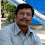### What is The Age? - Puzzle

My son Saji is 32 years younger than I and two years older than his sister.
If our combined age is 126,

what is the age of each of us?

1.son 47 and father 79

2.Saji 32 years , sister 30 years ,his 64 years

3.4.YOU COULNT EVEN GIVE THE ANSWER SAJI IS 32YRS, HIS SISTER IS 30YRS AND THEIR FATHER IS 64 YRS

5.6.7.x being Saji's age, x+(x+32)+(x-2)=126, so x=32, dad=64, sister=30.

8.Father is 64 Son is 32 daughter is 30 and their ages altogether comes out to 126

9.Father is 64 Son is 32 daughter is 30 and their ages altogether comes out to 126

10.son=32,daughter=30,father=64

11.take father age=x
saji(his son) age=y
his sister age=z

As per given information
x=32+y
z=y-2
x+y+z=126

After Solving these equation u can get
x=64 (Father's age)
y=32 (Son's Age)
z=30 (daughter's Age)

12.simply ...
lets say person telling this is "X"
: Saji = X-32
: Saji's Sis will be X-34

So,
X + (X-32) + (X-34) = 126

3X-66= 126

3X=192

So X = 64

Saji = 32 yrs

Saji's sister 30 yrs

13.14.15.father's age 64
saji's age 32
his sister ' age 30

16.17.Saji is 32. His sister is 30 and Dad is 64.

18.Saji is 32. His sister is 30 and Dad is 64.

19.Saji is 32. His sister is 30 and Dad is 64.

20.21.This comment has been removed by the author.

22.Parent = 64
Saji = 32
Sister = 30

23.Saji 32 ,sister 30,father64,

24.25.26.saji= x
father =x+32
sis= x-2

x+[x+32]+[x-2]=126
3x-2=126-32
3x=94+2
3x=96
x=96/3= 32
hence,saji's age= 32
father's age= 64
sister's age= 30

27.if "x" is father's age,

x+(x-32)+((x-32)-2)=126
x=64
son=32
daughter=30

28.Son is 32
Daughter is 30
Father is 64

29.Son is 32
Daughter is 30
Father is 64

30.father is 64 daughter 30 son 32

31.32.33.30, 32 and 64

34.his father age is 64 and sister age is 30(32-2=60,126-60=64)

35.36.Father- 64
Son-32
Daughter/ sister- 30

37.38.39.mom-64
son-32
sister-30

40.x+(x+34) = 126
x=46
Mom/Father Age = 80
Saji Age = 46

Saji's sister Age = 44